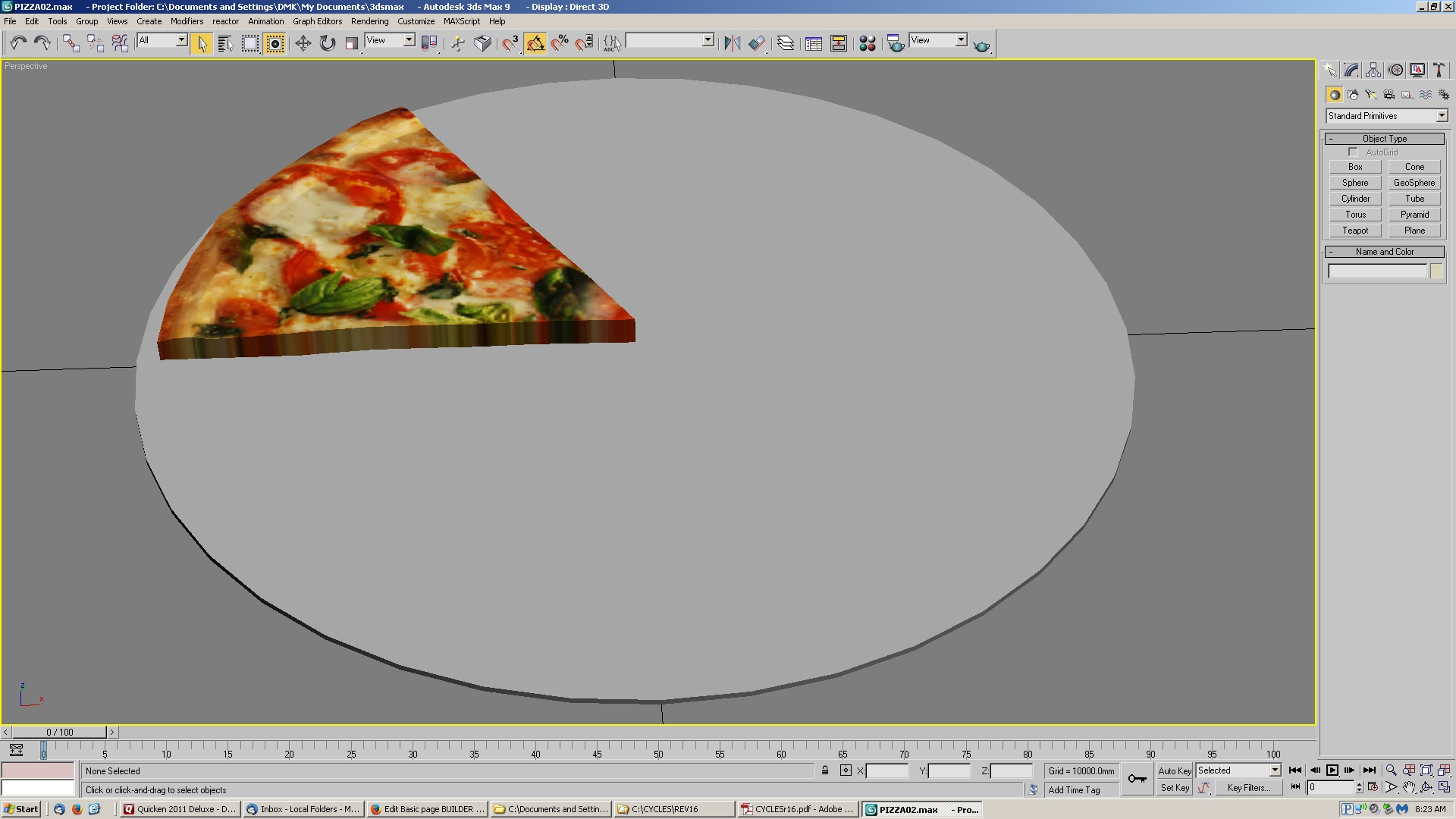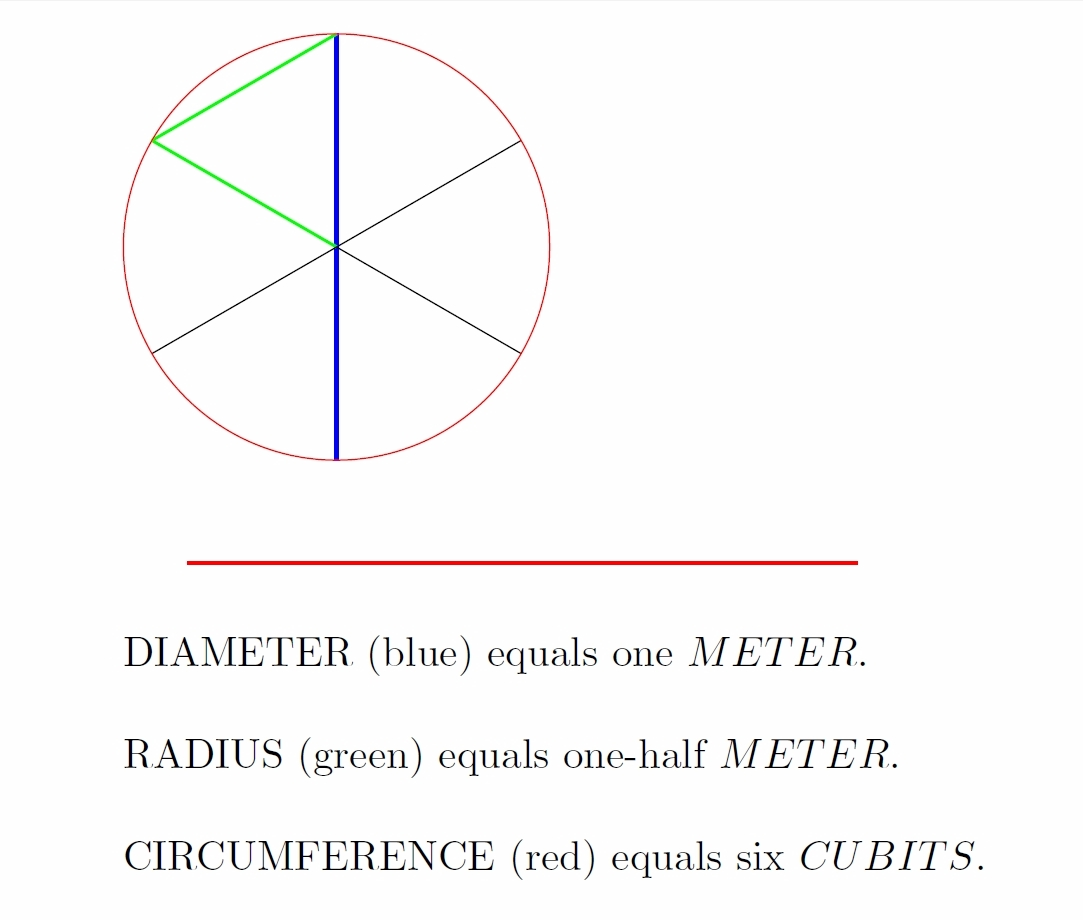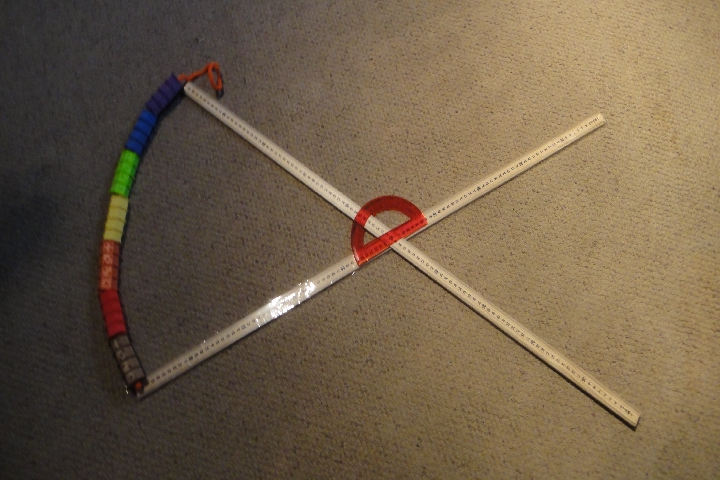# BUILDER UNITS - ROYAL CUBIT

Learning about this basic unit of the builders is easy as pie - pizza pie!

Start with a pizza pie one meter in diameter - just under 40 inches. That's a big pizza so slice it into six equal pieces.

Each piece is identical to all the others. Each piece consists of two straight edges and an arc of tasty crust.Question - how long is the arc of tasty crust?

Answer: 1 builder cubit!The meter and builder cubit are mathematically connected in this simple way.This relationship expressed in mathematical terms:

Each of the edges is half a meter in length, or 50 centimeters. Since

$DIAMETER=1m=100cm$

then

$RADIUS=\frac{DIAMETER}{2}=50cm$

$CIRCUMFERENCE = \pi*DIAMETER = \pi (1 meter)$

$CIRCUMFERENCE = 6 cubits$

$6 cubits = \pi meter$

$1 cubit = \frac{\pi}{6} meter$

$1 cubit ~= 0.5236m ~= 52.36cm$(photo courtesy Black Mountain Mill & Pizzeria)